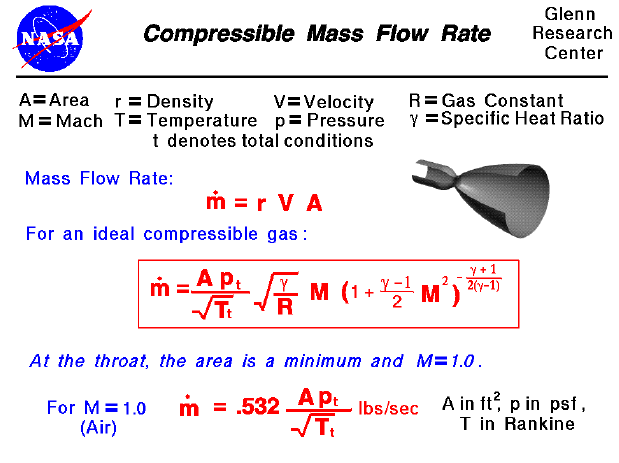A text only version of this slide is available which gives all of the flow equations.

The conservation of mass is a fundamental concept of physics. Within some problem domain, the amount of mass remains constant --mass is neither created or destroyed. The mass of any object is simply the volume that the object occupies times the density of the object. For a fluid (a liquid or a gas) the density, volume, and shape of the object can all change within the domain with time. And mass can move through the domain.

Mass Flow Rate

The conservation of mass (continuity) tells us that the mass flow rate through a tube is a constant and equal to the product of the density, velocity, and flow area. Considering the mass flow rate equation, it appears that for a given area, we can increase the mass flow rate to any desired point by increasing the velocity. However, in real fluids, compressibility effects limit the speed at which a flow can be forced through a given area.

Mass Flow Rate for an Ideal Compressible Gas

For a compressible, ideal gas, the mass flow rate is a unique function of the flow area, total pressure, temperature of the flow, properties of the gas, and the Mach number. This relationship is shown in the red box on this slide. To see the mathematical derivation of this equation, click here.

What does this equation tell us and how can we use this equation? If we had a flow through a tube at a fixed Mach number (fixed velocity), we could increase the mass flow rate through the tube by either increasing the area, increasing the total pressure, or decreasing the total temperature. Similarly, if we had a tube with a known area, we could increase the mass flow rate by increasing the total pressure or decreasing the total temperature. The effect of Mach number is a little hard to determine because the form of the Mach number term is fairly complex. Using calculus, we can determine that the maximum mass flow rate occurs when the Mach number is equal to one. To see the mathematical derivation of this equation, click here.

Mass Flow Rate at Nozzle Throat

If we have a tube with changing area, like the nozzle shown on the slide, the maximum mass flow rate through the system occurs when the Mach number is one at the smallest area. This location is called the throat of the nozzle, and the limit on the amount of mass flow rate that can be passed through the nozzle is called choking. The equation at the bottom of the slide gives some actual values for the maximum mass flow rate that can move through a nozzle throat. Going back to the mass flow rate equation in the box, if we know the throat area of a nozzle and know the mass flow rate, we can solve this equation for the Mach number (velocity) at any place in the nozzle, since the total pressure and temperature remain constant and only the area changes from place to place.

So with a nozzle, we can set the mass flow rate by setting the area of the throat. And we can set the exit Mach number by setting the area ratio from the throat to the exit. Using the isentropic relations, we can determine the pressure and temperature at the exit of the nozzle. And from the Mach number and temperature we can determine the exit velocity. If we consider the rocket thrust equation, we have now determined all the values necessary to determine the thrust of the rocket. You can explore the operation of a nozzle with our interactive nozzle simulator and design your own rockets!Guided Tours